# numpy.random.chisquare¶

numpy.random.chisquare(df, size=None)

Draw samples from a chi-square distribution.

When df independent random variables, each with standard normal distributions (mean 0, variance 1), are squared and summed, the resulting distribution is chi-square (see Notes). This distribution is often used in hypothesis testing.

Parameters : df : int Number of degrees of freedom. size : tuple of ints, int, optional Size of the returned array. By default, a scalar is returned. output : ndarray Samples drawn from the distribution, packed in a size-shaped array. ValueError : When df <= 0 or when an inappropriate size (e.g. size=-1) is given.

Notes

The variable obtained by summing the squares of df independent, standard normally distributed random variables: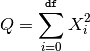is chi-square distributed, denoted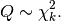The probability density function of the chi-squared distribution is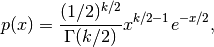where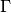is the gamma function,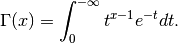References

NIST/SEMATECH e-Handbook of Statistical Methods

Examples

>>> np.random.chisquare(2,4)
array([ 1.89920014,  9.00867716,  3.13710533,  5.62318272])


#### Previous topic

numpy.random.binomial

#### Next topic

numpy.random.mtrand.dirichlet